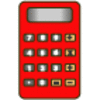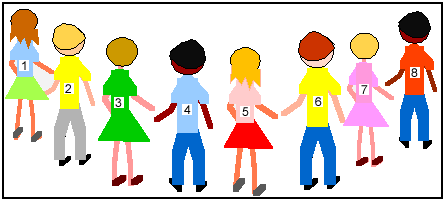#### You may also like### Biscuit Decorations

Andrew decorated 20 biscuits to take to a party. He lined them up and put icing on every second biscuit and different decorations on other biscuits. How many biscuits weren't decorated?### Constant Counting

You can make a calculator count for you by any number you choose. You can count by ones to reach 24. You can count by twos to reach 24. What else can you count by to reach 24?### Number Detective

Follow the clues to find the mystery number.

# Six in a Circle

## Six in a Circle

A class of $30$ children were numbered from $1$ to $30$. Here are some of the class:Their teacher set out a ring of $6$ chairs.

Child number $1$ sat on the first chair, child number $2$ on the second chair, child number $3$ on the third and so on going round the circle, up to child number $6$. The teacher then asked child number $7$ to stand behind child $1$, child $8$ to stand behind number $2$ etc. They continued this pattern until all the children were either sitting on a chair, or standing behind one.

How many people were standing behind each chair?
Who was standing behind child number $3$?
What would happen if there were only $4$ chairs?

### Why do this problem?

This problem is a useful one when children are being introduced to, or continuing work, on multiples (and factors). It could be illustrated practically with real chairs and children holding numbered cards.

### Key questions

Can you think of a good way to record this?
How about using counters numbered $1$ to $30$ or writing $1$ to $30$ on bits of paper and arranging them?
Can you think of a link to factors and multiples which may help?
Is $6$ a factor of $30$? Is $4$ a factor of $30$?

### Possible extension

Learners could try with other numbers besides $6$ and $4$. If they find these problems straightforward they could try this problem about multiples and other sets of numbers.

### Possible support

Many children will find it helpful to draw out the six chairs and use numbered counters (or numbered bits of paper) to find out what happens. Encourage quick recording before the bits of paper get blown away!